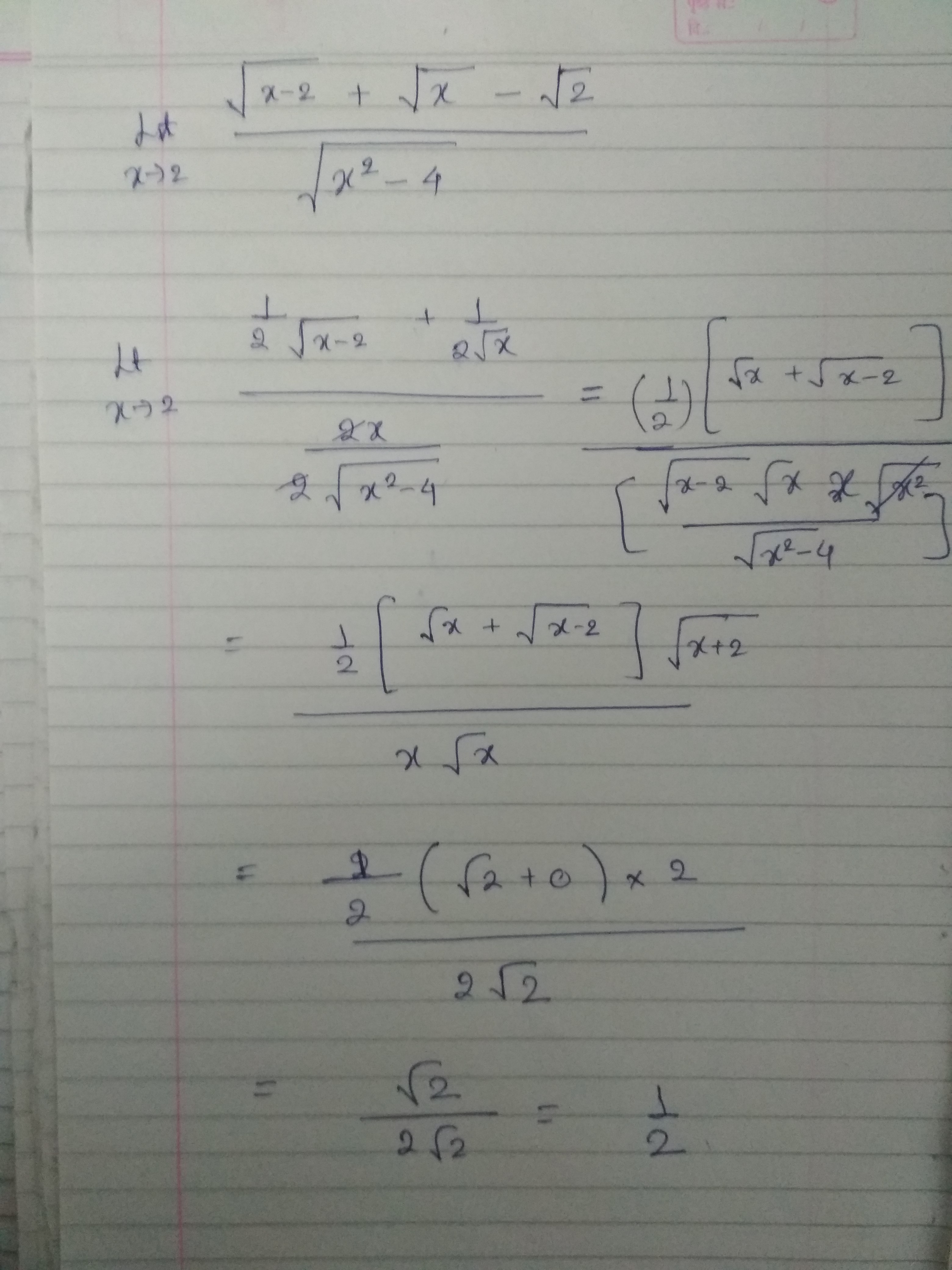×#### Thank you for registering.

One of our academic counsellors will contact you within 1 working day.

Click to Chat

1800-1023-196

+91-120-4616500

CART 0

• 0

MY CART (5)

Use Coupon: CART20 and get 20% off on all online Study Material

ITEM
DETAILS
MRP
DISCOUNT
FINAL PRICE
Total Price: Rs.

There are no items in this cart.
Continue Shopping
```

```
one year ago

```							hello feba, this is an ez ques.M= lim (√(x-2)+√x-√2)/√(x^2-4)= lim (√(x-2)+√x-√2)/√(x – 2)√(x+2)= [1/√(2+2)]*lim (√(x-2)+√x-√2)/√(x – 2)= ½ *lim (√(x-2)+√x-√2)/√(x – 2)= ½ *(lim 1 + (√x-√2)/√(x – 2))= ½ *[1+lim(√x-√2)/√(x – 2))]now to find L= lim(√x-√2)/√(x – 2)), multiply and divide by √x + √2 we getL= lim (x – 2)/[√(x – 2)(√x + √2)]= lim √(x – 2)/(√x + √2)= 0 at x tending to 2.hence, M= ½ = 0.5kindly approve :)
```
one year agoSaurabh Koranglekar
8397 Points
``````
one year ago
Think You Can Provide A Better Answer ?

## Other Related Questions on Differential Calculus

View all Questions »### Course Features

• 731 Video Lectures
• Revision Notes
• Previous Year Papers
• Mind Map
• Study Planner
• NCERT Solutions
• Discussion Forum
• Test paper with Video Solution### Course Features

• 51 Video Lectures
• Revision Notes
• Test paper with Video Solution
• Mind Map
• Study Planner
• NCERT Solutions
• Discussion Forum
• Previous Year Exam Questions